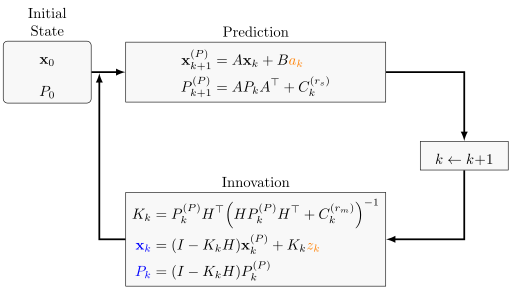# How does the error estimate change in the Kalman filter?

I am currently learning about the Kalman filter. A typical exam question is

In which steps does the uncertainty $P$ get bigger / smaller?

This is how I think the Kalman filter works / which notation I use:## Prediction step

For the prediction step, I would say it should increase:

In the prediction step, you have a matrix

$$\tilde{P} = A \cdot P \cdot A^T, \qquad A, P \in \mathbb{R}^{n \times n}$$

($A$ is the system matrix and $P$ is the estimate of the error.)

It has the property:

$$\det(\tilde{P}) = \det(A) \cdot \det(P) \cdot \det(A^T) = \det(A)^2 \cdot \det(P)$$

So if the determinant of $P$ is how we say if it gets bigger, then it will get bigger in the prediction step if $\det(A) > 0.5$. Otherwise, it might still get bigger as the system noise $C_{k}^{(s)}$ gets added.

## Filter step

I have no clue what I can say in the filter step:

\begin{align} \det((I- K_k H) P_k^{(P)}) &= \det(I- K_k H) \cdot \det(P_k^{(P)})\\ &= \det(I P_k^{(P)}- K_k H P_k^{(P)})\\ \end{align}

• I think that question would be better asked at the dsp stackexchange. – Emiswelt Jul 7 '16 at 9:48

## 1 Answer

First of all $det(A) det(A^T) = det(A)^2$.

I do not think that measuring the determinant of $P$ is a good index for checking the uncertainty of your states. A very small determinant is telling you that at least of the eigenvalues of $P$ is close to zero, but others might be big. If you have a two dimensional system, your ellipse might have one very small axis and the other one might be very big.

Since you have here an autonomous system, if your system is stable you know that $x$ will approach zero, so you do not need any observation in order to make your $P$ smaller.

When you make an observation, the term $KH$ is positive semidefinite, so $P_{k+1} < (I-KH)P_K$